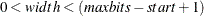### UnpackBits Function

`r = UnpackBits` ( source, start, width ) ;

The UnpackBits call retrieves bit values from a packed integer array. The inputs to the UnpackBits function are as follows:

 source is an array containing the packed bit values. start is the starting bit, with the lowest bit starting at 1. width is the number of bits to retrieve. A value of 1 retrieves a single bit.

The UnpackBits function facilitates the extraction of bit values from arbitrary locations in an integer array. One common use for it is to retrieve bit values from an integer solution segment in a user objective or genetic operator routine. The return value, start, and width parameters are consistent with the PackBits call, which you can use to store bit values to an integer array.

The start parameter is the lowest desired bit position in the bit vector, corresponding to the least significant bit of the return value. The start parameter can range in value from 1 to maxbits, where maxbits is the product of 32 times the number of elements in the integer array.

The width parameter is the number of bits to be read into the return value from the array. It is bounded by, and must also not exceed 32.